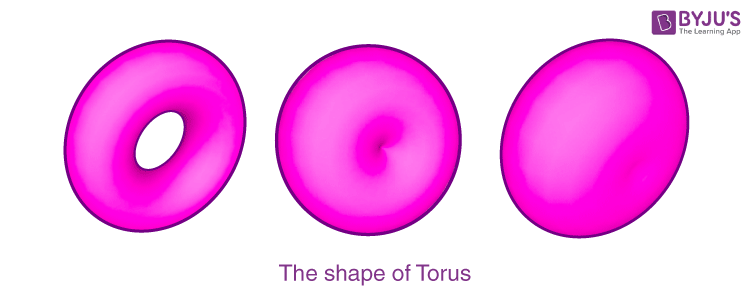# Toric Lens

## What is Toric Lens?

A lens in which the focal length and optical power are different in two different directions at 90 degrees with respect to each other is known as a toric lens. One side looks like a torus’s cap whereas the other side is generally spherical. It’s used mostly in eye care i.e. with the intraocular lens for correcting astigmatism, in contact lenses, and in eyeglasses.

## What is Torus?To understand what a torus is we will have to visualize it. There is a circle with a radius of r that’s rotating in the axis that lies in the same plane and from the center of the circle is at a distance of R. If R is bigger than r then a torus of the shape of a ring will be produced. If R is equal to r then the opening gets reduced to point and a horn torus is created. If R is less than r, a spindle torus is created where the opening is reduced down to two dips. When R is zero, the torus gets degenerated into a sphere of radius r.

## Radius of Curvature & Optical Power:

The largest radius of curvature of the surface of a toric lens is R+r, which can be equated to the smallest refractive power which is S, represented as,

$$\begin{array}{l}S=\frac{n-1}{R+r}\end{array}$$

The lens’s index of refraction is n in the equation.

The vice versa is also true i.e. when the radius is the smallest the refractive power is at the greatest. This gives us,

$$\begin{array}{l}S=\frac{n-1}{r}\end{array}$$

This concept is proof of the lens’s cylindrical power combined with the spherical power of another facet.

## Ray of Light & its Refractive Power:

A ray of light that strikes the torus on an x, y plane suffers refraction in consideration of the largest radius of curvature. Thus, as we discussed before it will have the least refractive power. Whereas the ray of light that enters through the z-axis (axis of revolution) suffers refraction in accordance with the least value of the radius of curvature which in turn makes it have the highest power of refraction.

Placed at 90 degrees to each other this lens has two refractive powers due to the above properties. If the light enters through intermediate angles between these two axes the value of refractive power changes from higher to a smaller value or vice versa, in turn, compensating for the eye defects.

Modern technology with its computer-controlled designing skills has created a toric lens that works on the concept of achieving better vision correction through deviating from the toric shape.

## Frequently Asked Questions – FAQs

Q1

### What is a lens?

A lens is a piece of transparent glass which concentrates or disperses light rays when passes through them by refraction.
Q2

### What is a toric lens?

A lens in which the focal length and optical power are different in two different directions at 90 degrees with respect to each other is known as a toric lens.

Q3

### How does a toric lens appear?

The toric lens appears like a torus’s cap whereas the other side is generally spherical.
Q4

### What is the formula when the radius is the smallest the refractive power is at the greatest?

$$\begin{array}{l}S=\frac{n-1}{r}\end{array}$$
.
Q5

### Where is the toric lens used?

The toric lens is used in the eye care field along with the intraocular lens for correcting astigmatism in contact lenses, and in eyeglasses.
Test your Knowledge on Toric Lens